Next: Linear Problems with Prolog Up: Adding Computation Domains Previous: Domains

# Linear (Dis)Equations

Linear (dis)equations were, in practice, pioneered by the CLP(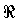) system, which offered a Prolog-like interface, where arithmetical symbols were enriched to express constraints. Other implementations (namely, Prolog IV, CHIP, SICStus ...) have chosen to implement this constraint system as well, as it has a well-known solving procedure, and is useful in a range of applications. We will use Prolog IV in the examples, as it is a quite reasonably known logic programming system, and it has several constraint systems available.

A note on syntax: CLP systems tend to have some variations in the syntax of similar operations. This may be slightly confusing at first, but it is not a real problem: different syntaxes are easily understood, once the underlying language design principles are known.

We will augment the language with the following components:

• Numbers, both integers and floating point numbers (which aproximate real numbers), written as usual. Addiotinally, expressions like 3.7e5 represent the number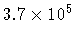.
• Arithmetic operators (+, -, *, /) , written in the usual infix form. They allow us to construct arithmetic terms, using numbers and variables: 3 + 4, X + 3 * (6 - Y). Those arithmetic terms stand for the corresponding arithmetic expressions.
• The constraint =/2, which now stands for arithmetical equality: two expressions are now said to be equal if they can be arithmetically reduced to the same expression.
• More arithmetical constraints, which act as the corresponding arithmetical relational operators:

Prolog IV name Arithmetical meaning
gelin(X, Y)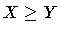gtlin(X, Y) X > Y
lelin(X, Y)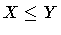ltlin(X, Y) X < Y

Note that all of them have the suffix lin, which stands for linear: the reason for that will become clear later.

Prolog IV (and other CLP languages) can solve equations directly typed in the top-level prompt. These examples are taken from a Prolog IV session:

?- 4 - Y = 3.
Y = 1
?- X = 3*Y - X/2, X+Y = Y - 4*X + 7.
Y = 7/10, X = 7/5.


(The prompt of the Prolog IV interpreter is >>, but we will be using ?- throughout this paper). By default, answers are returned as fractions because Prolog IV uses infinite precision arithmetic when possible. The equations above had a unique solution, but equations may have no solutions:

?- X = 3*Y - X/2, X+Y = Y - 4*X + 7, lelin(X, Y).
false.


And sometimes there exists an infinite number of solutions:

?- X = Y + 3.
X ~ real, Y ~ real


which means that X and Y are just real numbers. The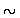syntax needs further explanation, but we will delay it until later: it will suffice by now to understand it as meaning belongs to the class of''--in this case, X belongs to the class of the real numbers''.

Prolog IV does not output complex relationships, although it is of course aware of them. In the previous example, Yreal, Xreal is actually a weak answer, for the constraint X + Y = 3 is known by the system. A clearer example is the one below:

Example 3.2   The following query represents the constraint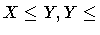X, from which X = Y can be deduced:

?- gelin(X, Y), gelin(Y, X).
Y ~ real, X ~ real


No output of constraints is given, but the solver is internally aware of the constraint X = Y.

?- gelin(X, Y), gelin(Y, X), X = 4.
Y = 4, X = 4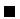There is a problem in generating output for complex answer constraints: the constraint system, after simplification, has to be projected onto the query variables (because these are the ones the user knows about), and this is not easy (or even feasible) in some constraint domains.Next: Linear Problems with Prolog Up: Adding Computation Domains Previous: Domains
MCL
1998-12-03